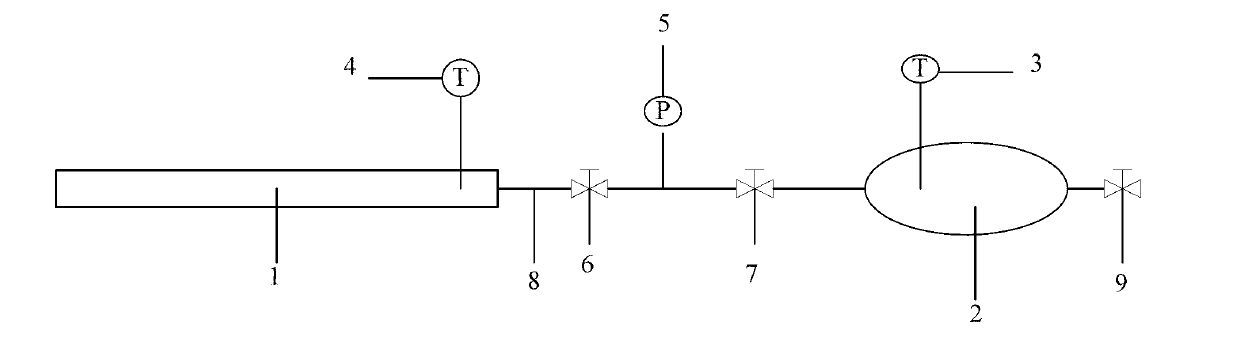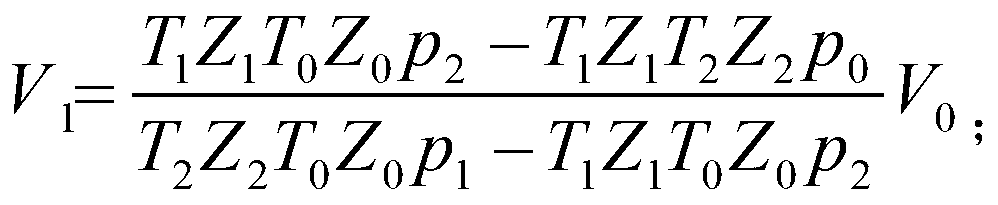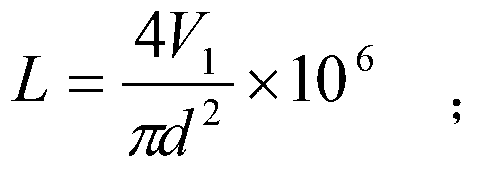# Measuring device blocking position of gas pipeline and measuring method thereof

## A technology for gas pipelines and measuring devices, applied in gas/liquid distribution and storage, pipeline systems, mechanical equipment, etc., can solve the problems of time-consuming and laborious staff experience, increased production costs, and higher requirements.

Inactive Publication Date: 2013-09-04
XI'AN POLYTECHNIC UNIVERSITY
4 Cites 13 Cited by

## AI-Extracted Technical Summary

### Problems solved by technology

 The former has produced great benefits through the application of gas fields, but there is a problem of a sharp increase in the consumption of methanol. At the same time, the treatment of alcohol-containing sewage also requires additional specia...
View more

## Abstract

The invention discloses a measuring device of a blocking position of a gas pipeline. The measuring device comprises a gas tank, and a first Pt thermometer and an exhaust pressure-resistance valve are connected to the gas tank. The gas tank is connected with the gas pipeline through a pressure-resistance flexible pipe, a second Pt thermometer is arranged on the gas pipeline, and a first pressure-resistance valve and a second pressure-resistance valve are respectively arranged on the pressure-resistance flexible pipe. A pressure sensor is connected to the pressure-resistance flexible pipe between the two pressure-resistance valves. The invention further discloses a measuring method of the measuring device. The measuring method comprises the steps that firstly, the initial pressure and temperature of gas in the gas tank are measured; secondly, the initial pressure and temperature of gas in the gas pipeline are measured; thirdly, the two pressure-resistance valves are opened, the gas in the gas pipeline flows towards the gas tank, and the pressure and temperature of the gas in a balanced state are measured; fourthly, the two pressure-resistance valves are closed, and the exhaust pressure-resistance valve of the gas tank is opened to discharge the gas; fifthly, an algorithm module in a computer is utilized to obtain the blocking position of the gas pipeline. The measuring device of the blocking position of the gas pipeline and the measuring method of the measuring device can accurately determine the blocking position of the gas pipeline.

Application Domain

Pipeline systems

Technology Topic

Gas pipelineThermometer +4

## Image

•••## Examples

• Experimental program(4)

### Example Embodiment

 Example 1
 The simulated gas in gas pipeline 1 is methane CH 4 , Where the critical parameter of methane is known as: p c =4596kPa, T c =190.53K;
 The initial pressure value p of methane gas in the gas pipeline is measured by the pressure sensor 5 1 200.0kPa;
 At room temperature 20℃, with inner diameter d=80mm, L s =9.10m seamless steel pipe is gas pipeline 1, with V 0 =0.11m 3 The oval seamless steel tank is the gas tank 2, and the gas tank 2 is equipped with an exhaust pressure valve 9. Use the air in gas tank 2 with methane CH in gas line 1. 4 out;
 Close the exhaust pressure valve 9 and measure the initial pressure value p of the methane gas in the gas tank 2 with the pressure sensor 5 with an accuracy of 0.1kPa 0 , Use the first Pt thermometer 3 to measure the initial gas temperature T in the gas tank 2 0 , The initial pressure value p of methane in the gas tank 2 is measured 0 =100kPa; the temperature in the gas tank 2 is measured as room temperature T 0 =293.15K;
 Close the second pressure valve 7 and open the first pressure valve 6, use the pressure sensor 5 to measure the initial pressure of the methane gas in the gas pipeline 1. The initial pressure of the methane gas in the gas pipeline 1 is p 1 =200.0kPa, the initial temperature of methane gas in gas pipeline 1 is room temperature T 1 =293.15K;
 The first pressure valve 6 and the second pressure valve 7 between the gas tank 2 and the gas pipeline 1 are all opened, the methane gas in the gas pipeline 1 flows into the gas tank 2, and the pressure of the methane gas in the gas pipeline 1 is The gas pressure in tank 2 reaches equilibrium, the pressure gauge of pressure sensor 5 shows that the data is stable, and pressure sensor 5 shows that the pressure when the gas reaches equilibrium is p 2 =159.3kPa, the temperature at equilibrium is room temperature T 2 =293.15K;
 Close the first pressure-resistant valve 6 and the second pressure-resistant valve 7, open the exhaust pressure-resistant valve 9 on the gas tank 2, and release the gas in the gas tank 2;
 Set the initial pressure value of methane gas in gas tank 2 p 0 And initial temperature value T 0 , The initial pressure value of methane gas in gas pipeline 1 p 1 And initial temperature value T 1 , The pressure value p of the equilibrium state of methane gas 2 And temperature value T 2 Enter the computer and use the algorithm module to perform the following calculations:
 The corresponding comparison parameter is p 0r =p 0 /p c =0.022,T 0r =T 0 /T c =1.539, generalized compression factor graph based on two parameters, Z 0 =f(p 0r ,T 0r )=1;
 The corresponding comparison parameter is p 1r =p 1 /p c =0.044, T 1r =T 1 /T c =1.539, according to the two-parameter generalized compression factor graph, Z 1 =f(p 1r ,T 1r )=1;
 The corresponding comparison parameter is p 2r =p 2 /p c =1.221, T 2r =T 2 /T c =1.539, generalized compression factor graph based on two parameters, Z 2 =f(p 2r ,T 2r )=1.
 The initial gas pressure value p in the gas tank 2 obtained above 0 , The initial gas temperature value T in the gas tank 0 、The compressibility factor Z of the gas in the gas tank in the initial state 0 , The initial pressure value of the gas in the gas pipeline p 1 , The initial gas temperature value T in the gas pipeline 1 、The compressibility factor Z of the gas in the gas pipeline in the initial state 1 , The balance pressure value p after connecting the gas pipeline and the gas tank 2 , The gas equilibrium temperature value T after the gas pipeline and the gas tank are connected 2 , Gas pipeline and gas tank connection after equilibrium gas compression factor Z 2 , The volume of the gas tank V 0 , Substitute the inner diameter d of the gas pipeline V 1 = T 1 Z 1 T 0 Z 0 p 2 - T 1 Z 1 T 2 Z 2 p 0 T 2 Z 2 T 0 Z 0 p 1 - T 1 Z 1 T 0 Z 0 p 2 V 0 with L = 4 V 1 πd 2 X 10 6 ;
 After calculation:
 L=9.07m, relative error: E%=(measured value-actual value)/actual value=(L-L s )/L s =(9.07-9.10)m/9.10m=-0.3%.

### Example Embodiment

 Example 2
 The simulated gas in gas pipeline 1 is methane CH 4 , Where the critical parameter of methane is known as: p c =4596kPa, T c =190.53K;
 The initial pressure value p measured by the pressure sensor 5 1 Is 8000.0kPa;
 At room temperature 20℃, with inner diameter d=80mm, L s =9.10m seamless steel pipe is a gas pipeline, with V 0 =0.11m 3 The oval seamless steel tank is the gas tank 2, and the gas tank 2 is equipped with an exhaust pressure valve 9. Use the air in gas tank 2 with methane CH in gas line 1. 4 out;
 Close the exhaust pressure valve 9 and measure the initial pressure value p of the methane gas in the gas tank 2 with the pressure sensor 5 with an accuracy of 0.1kPa 0 , Use the first Pt thermometer 3 to measure the initial gas temperature T in the gas tank 2 0 , The initial pressure value p of methane in the gas tank 2 is measured 0 =100kPa, the temperature in the gas tank 2 is measured to be room temperature T 0 =293.15K;
 Close the second pressure valve 7 and open the first pressure valve 6, use the pressure sensor 5 to measure the initial pressure of the methane gas in the gas pipeline 1. The initial pressure of the methane gas in the gas pipeline 1 is p 1 =8000.0kPa, the initial temperature of methane gas in gas pipeline 1 is room temperature T 1 =293.15K;
 The first pressure valve 6 and the second pressure valve 7 between the gas tank 2 and the gas pipeline 1 are all opened, the methane gas in the gas pipeline 1 flows into the gas tank 2, and the pressure of the methane gas in the gas pipeline 1 is The gas pressure in tank 2 reaches equilibrium, the pressure gauge of pressure sensor 5 shows that the data is stable, and pressure sensor 5 shows that the pressure when the gas reaches equilibrium is p 2 =5335.2kPa, the temperature at equilibrium is room temperature T 2 =293.15K;
 Set the initial pressure value of methane gas in gas tank 2 p 0 And initial temperature value T 0 , The initial pressure value of methane gas in gas pipeline 1 p 1 And initial temperature value T 1 , The pressure value p of the equilibrium state of methane gas 2 And temperature value T 2 Enter the computer and use the calculation model to perform the following calculations:
 The corresponding comparison parameter is p 1r =p 1 /p c =1.741, T 1r =T 1 /T c =1.539, according to the two-parameter generalized compression factor graph, Z 1 =f(p 1r ,T 1r )=0.85;
 The corresponding comparison parameter is p 0r =p 0 /p c =0.022,T 0r =T 0 /T c =1.539, generalized compression factor graph based on two parameters, Z 0 =f(p 0r ,T 0r )=1;
 The corresponding comparison parameter is p 2r =p 2 /p c =1.161, T 2r =T 2 /T c =1.539, generalized compression factor graph based on two parameters, Z 2 =f(p 2r ,T 2r )=0.95.
 The initial pressure value p of the gas in the gas tank obtained above 0 , The initial gas temperature value T in the gas tank 0 、The compressibility factor Z of the gas in the gas tank in the initial state 0 , The initial pressure value of the gas in the gas pipeline p 1 , The initial gas temperature value T in the gas pipeline 1 、The compressibility factor Z of the gas in the gas pipeline in the initial state 1 , The balance pressure value p after connecting the gas pipeline and the gas tank 2 , The gas equilibrium temperature value T after the gas pipeline and the gas tank are connected 2 , Gas pipeline and gas tank connection after equilibrium gas compression factor Z 2 , The volume of the gas tank V 0 , Substitute the inner diameter d of the gas pipeline V 1 = T 1 Z 1 T 0 Z 0 p 2 - T 1 Z 1 T 2 Z 2 p 0 T 2 Z 2 T 0 Z 0 p 1 - T 1 Z 1 T 0 Z 0 p 2 V 0 with L = 4 V 1 πd 2 X 10 6 ;
 After calculation, L=9.05m, relative error: E%=-0.6%.

### Example Embodiment

 Example 3
 The simulated gas in gas pipeline 1 is carbon dioxide CO 2 , Where the critical parameter of carbon dioxide is known as: p c =7375kPa, T c =304.13K;
 The initial pressure value p of the carbon dioxide gas in the gas pipeline is measured by the pressure sensor 5 1 200.0kPa;
 At room temperature 20℃, with inner diameter d=80mm, L s =9.10m seamless steel pipe is simulated gas pipeline 1, with V 0 =0.11m 3 The oval seamless steel tank is the gas tank 2, and the gas tank 2 is equipped with an exhaust pressure valve 9. Use the simulated gas carbon dioxide CO for the air in the gas tank 2 2 out;
 Close the exhaust pressure valve 9 and measure the initial pressure value p of the carbon dioxide gas in the gas tank 2 with the pressure sensor 5 with an accuracy of 0.1kPa 0 , Use the first Pt thermometer 3 to measure the initial gas temperature T in the gas tank 2 0 , The initial pressure value p of carbon dioxide in the gas tank 2 is measured 0 =100kPa; the temperature in the gas tank 2 is measured as room temperature T 0 =293.15K;
 Close the second pressure valve 7 and open the first pressure valve 6, use the pressure sensor 5 to measure the initial pressure of carbon dioxide gas in the gas pipeline 1. The initial pressure of carbon dioxide gas in the gas pipeline 1 is p 1 =200.0kPa, the initial temperature of carbon dioxide gas in gas pipeline 1 is room temperature T 1 =293.15K;
 Open the first pressure valve 6 and the second pressure valve 7 between the gas tank 2 and the gas pipeline 1, the carbon dioxide gas in the gas pipeline 1 flows into the gas tank 2, and the pressure of the carbon dioxide gas in the gas pipeline 1 is The gas pressure in tank 2 reaches equilibrium, the pressure gauge of pressure sensor 5 shows that the data is stable, and pressure sensor 5 shows that the pressure when the gas reaches equilibrium is p 2 =159.0kPa, the temperature at equilibrium is room temperature T 2 =293.15K;
 Close the first pressure-resistant valve 6 and the second pressure-resistant valve 7, open the exhaust pressure-resistant valve 9 on the gas tank 2, and release the gas in the gas tank 2;
 Set the initial pressure value of methane gas in gas tank 2 p 0 And initial temperature value T 0 , The initial pressure value of methane gas in gas pipeline 1 p 1 And initial temperature value T 1 , The pressure value p of the equilibrium state of methane gas 2 And temperature value T 2 Enter the computer and use the calculation model to perform the following calculations:
 The corresponding comparison parameter is p 1r =p 1 /p c =0.027, T 1r =T 1 /T c =0.964, generalize the compression factor graph Z according to two parameters 1 =f(p 1r ,T 1r )=1;
 The corresponding comparison parameter is p 0r =p 0 /p c =0.014,T 0r =T 0 /T c =0.964, generalized compression factor graph based on two parameters, Z 0 =f(p 0r ,T 0r )=1;
 The corresponding comparison parameter is p 2r =p 2 /p c =0.022, T 2r =T 2 /T c =0.964, generalized compression factor graph based on two parameters, Z 2 =f(p 2r ,T 2r )=1;
 The initial pressure value p of the gas in the gas tank obtained above 0 , The initial gas temperature value T in the gas tank 0 、The compressibility factor Z of the gas in the gas tank in the initial state 0 , The initial pressure value of the gas in the gas pipeline p 1 , The initial gas temperature value T in the gas pipeline 1 、The compressibility factor Z of the gas in the gas pipeline in the initial state 1 , The balance pressure value p after connecting the gas pipeline and the gas tank 2 , The gas equilibrium temperature value T after the gas pipeline and the gas tank are connected 2 , Gas pipeline and gas tank connection after equilibrium gas compression factor Z 2 , The volume of the gas tank V 0 , Substitute the inner diameter d of the gas pipeline V 1 = T 1 Z 1 T 0 Z 0 p 2 - T 1 Z 1 T 2 Z 2 p 0 T 2 Z 2 T 0 Z 0 p 1 - T 1 Z 1 T 0 Z 0 p 2 V 0 with L = 4 V 1 πd 2 X 10 6 ;
 After calculation, L=9.07m, relative error: E%=-0.3%.

## PUM## Description & Claims & Application Information

We can also present the details of the Description, Claims and Application information to help users get a comprehensive understanding of the technical details of the patent, such as background art, summary of invention, brief description of drawings, description of embodiments, and other original content. On the other hand, users can also determine the specific scope of protection of the technology through the list of claims; as well as understand the changes in the life cycle of the technology with the presentation of the patent timeline. Login to view more.
Who we serve
• R&D Engineer
• R&D Manager
• IP Professional
Why Eureka